# Bicycle

The bicycle pedal gear has 36 teeth, the rear gear wheel has 10 teeth. How many times turns rear wheel, when pedal wheel turns 120x?

Result

n =  432

#### Solution:Leave us a comment of example and its solution (i.e. if it is still somewhat unclear...):

Showing 0 comments:Be the first to comment!## Next similar examples:

1. Seeds 2How many seeds germinated from 1000 pcs, when 23% no emergence?
2. DisjointHow many elements have union and intersection of two disjoint sets when the first have 1 and secodn 8 elements.
3. Chocolate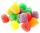I eat 24 chocolate in 10 days. How many chocolate I eat in 15 days at the same pace?
4. Cost reductionWindbreaker cost after discounted SKK 1275, which were 3/4 of original price. How many SKK originally cost windbreaker? How many SKK was cost reduction?
5. Liquid soap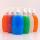Liquid soap lasted two weeks to one member of the family. How many days will last, if it use: a) two members b) 4 members c) seven members?
6. Obtuse angleWhich obtuse angle is creating clocks at 17:00?
7. Reciprocal equation 3Solve reciprocal equation: 1/2 + 2/3=1/x
8. IronIron ore contains 57% iron. How much ore is needed to produce 20 tons of iron?
9. Combine / add termCombine like terms 4c+c-7c
10. Simple equationSolve for x: 3(x + 2) = x - 18
11. AverageThe arithmetic mean of the two numbers is 71.7. One number is 5. Calculate the second number.
12. ClassIn 7.C clss are 10 girls and 20 boys. Yesterday was missing 20% of girls and 50% boys. What percentage of students missing?
13. Bronze medal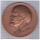To produce 1 kg of bronze must be 0.95 kg copper, 0.04 kg of tin, 0.01 kg zinc. The bronze medal has a weight of 8.5 g. How much copper, tin and zinc does medal have?
14. Field on plan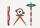Plan has a scale of 1: 2500.Determine dimensions in centimeters which will have on plan field with a length of 310 meters and a width of 182.5 meters.
15. HotelThe hotel has a p floors each floor has i rooms from which the third are single and the others are double. Represents the number of beds in hotel.
16. ClassIn a class are 32 pupils. Of these are 8 boys. What percentage of girls are in the class?
17. Average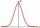Average of 7 numbers is 65. What is its sum?